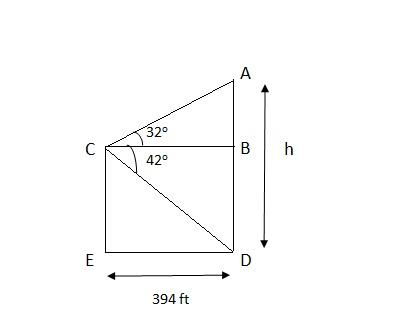# A radio tower is located 394 feet from a building. From a window in the building, a person...

## Question:

A radio tower is located 394 feet from a building. From a window in the building, a person determines that the angle of elevation to the top of the tower is 32 degrees and that the angle of depression to the bottom of the tower is 42 degrees. How tall is the tower?

## Trigonometry:

The problems of trigonometry related to triangles, where height, angle of elevation or depression need to calculated are simple. Here trigonometric ratios of base, perpendicular and hypotenuse are extensively used.

## Answer and Explanation:

Let us first draw the diagram for given problem:Given:

{eq}\begin{align*} & ED= 394 feet \\ \\ & \angle{ACB}= 32 \ degree \\ \\ &...

See full answer below.

Become a Study.com member to unlock this answer! Create your account

#### Learn more about this topic:

What is Trigonometry? - Functions, Formulas & Applications

from High School Trigonometry: Homework Help Resource

Chapter 22 / Lesson 11
32K